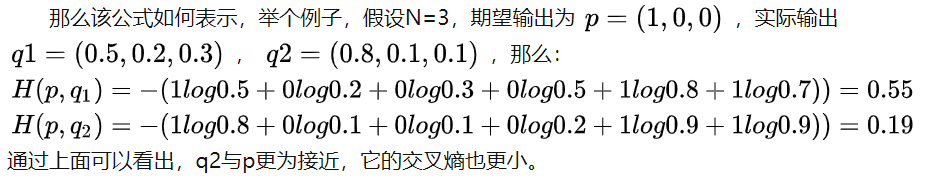# 【Pytorch】交叉熵损失函数 CrossEntropyLoss() 详解

### 文章目录

• 一、损失函数 nn.CrossEntropyLoss()
• 二、什么是交叉熵
• 三、Pytorch 中的 CrossEntropyLoss() 函数
• 参考链接

• # 一、损失函数 nn.CrossEntropyLoss()

交叉熵损失函数 nn.CrossEntropyLoss() ，结合了 nn.LogSoftmax() 和 nn.NLLLoss() 两个函数。

它在做分类（具体几类）训练的时候是非常有用的。

# 二、什么是交叉熵

交叉熵主要是用来判定 实际的输出与期望的输出的接近程度

举个例子：在做分类训练的时候，如果一个样本属于第 K 类，那么这个类别所对应的输出节点的输出值应该为1，而其他节点的输出都为0，即 [0,0,1,0,….0,0] ，这个数组也就是样本的 Label，是神经网络最期望的输出结果。我们用交叉熵损失函数来衡量网络的实际输出与正确标签的差异，利用这种差异经过反向传播去更新网络参数。

交叉熵：它主要刻画的是实际输出（概率）与期望输出（概率）的距离，也就是交叉熵的值越小，两个概率分布就越接近。假设概率分布p为期望输出，概率分布q为实际输出，则交叉熵定义为：实际例子：# 三、Pytorch 中的 CrossEntropyLoss() 函数

Pytorch中计算的交叉熵并不是采用第 “二” 节中的公式计算得到的，而是采用交叉熵的另外一种方式计算得到的：Pytorch中CrossEntropyLoss()函数的主要是将softmax-log-NLLLoss合并到一块得到的结果。

1. Softmax后的数值都在0~1之间，所以 ln 之后值域是负无穷到0。
2. 然后将Softmax之后的结果取log，将乘法改成加法减少计算量，同时保障函数的单调性 。
3. NLLLoss 的结果就是把上面的输出与 Label 对应的那个值拿出来，去掉负号，再求均值。

一个实例：直接使用pytorch中的 nn.CrossEntropyLoss() 计算得到的结果与 softmax-log-NLLLoss计算得到的结果是一致的

``````import torch
import torch.nn as nn

x_input = torch.randn(3, 3)  # 随机生成输入
print('x_input:\n', x_input)
y_target = torch.tensor([1, 2, 0])  # 设置输出具体值 print('y_target\n',y_target)

# 计算输入softmax，此时可以看到每一行加到一起结果都是1
softmax_func = nn.Softmax(dim=1)
soft_output = softmax_func(x_input)
print('soft_output:\n', soft_output)

# 在softmax的基础上取log
log_output = torch.log(soft_output)
print('log_output:\n', log_output)

# 对比softmax与log的结合与nn.LogSoftmaxloss(负对数似然损失)的输出结果，发现两者是一致的。
logsoftmax_func=nn.LogSoftmax(dim=1)
logsoftmax_output=logsoftmax_func(x_input)
print('logsoftmax_output:\n', logsoftmax_output)

# pytorch中关于NLLLoss的默认参数配置为：reducetion=True、size_average=True
nllloss_func = nn.NLLLoss()
nlloss_output = nllloss_func(logsoftmax_output, y_target)
print('nlloss_output:\n', nlloss_output)

# 直接使用pytorch中的loss_func=nn.CrossEntropyLoss()看与经过NLLLoss的计算是不是一样
crossentropyloss = nn.CrossEntropyLoss()
crossentropyloss_output = crossentropyloss(x_input, y_target)
print('crossentropyloss_output:\n', crossentropyloss_output)
``````

输出结果：

``````x_input:
tensor([[ 0.3321,  0.5043, -1.1833],
[-2.1702, -1.8563,  1.0706],
[ 1.7341,  0.2554, -1.3358]])
soft_output:
tensor([[0.4153, 0.4934, 0.0913],
[0.0358, 0.0490, 0.9152],
[0.7847, 0.1789, 0.0364]])
log_output:
tensor([[-0.8787, -0.7064, -2.3940],
[-3.3295, -3.0155, -0.0886],
[-0.2424, -1.7212, -3.3123]])
logsoftmax_output:
tensor([[-0.8787, -0.7064, -2.3940],
[-3.3295, -3.0155, -0.0886],
[-0.2424, -1.7212, -3.3123]])
nlloss_output:
tensor(0.3458)
crossentropyloss_output:
tensor(0.3458)
``````

来源：想变厉害的大白菜